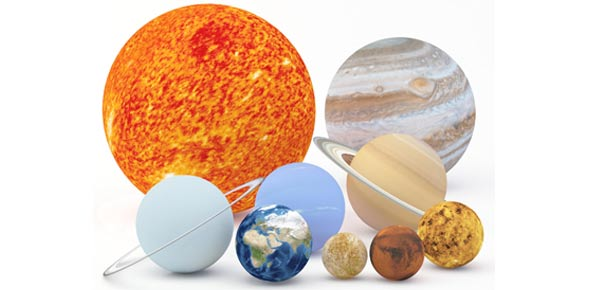# General Information On The Astronomy Quiz 15

14 Questions | Total Attempts: 107SettingsThe quick quiz below is the fifteenth one is a series of tests aimed at testing your general understanding on astronomy. It is very interesting and covers a lot of the aspects about the universe you have learnt in various classes. Give it a shot and all the best of luck!

• 1.
Information comes to us from the Sun in the form of:
• A.

Infrared waves

• B.

Visible (light) waves

• C.

Ultraviolet waves

• D.

All of the above

• E.

None of the above

• 2.
Light waves are most often labeled using their:
• A.

Amplitude

• B.

Power

• C.

Wavelength and/or frequency

• D.

All of the above

• E.

None of the above

• 3.
The wavelength and the frequency of a wave are related by:
• A.

The color of light

• B.

The speed of light

• C.

The light power

• D.

All of the above

• E.

None of the above

• 4.
The speed of light, "c", is:
• A.

A constant only within our solar system

• B.

A constant only within our galaxy

• C.

A constant everywhere in our universe

• D.

Not constant at all, it depends on the frequency of the light

• E.

None of the above

• 5.
The temperature of the Sun can be determined if we measure the solar spectrum(light intensity vs wavelength) and we compared it to:
• A.

Einstein's theory of special relativity

• B.

Kepler's laws

• C.

The spectral distribution for "black bodies"

• D.

The spectral distribution for "white hot bodies"

• E.

None of the above

• 6.
The mass of the Sun can be determined once we know:
• A.

Newton's laws of gravitational motion

• B.

Kepler's laws

• C.

The actual radar distance between Earth and Venus

• D.

All of the above

• E.

None of the above

• 7.
In addition to the Earth to Sun distance in meters, what is needed to measure the mass of the Sun:
• A.

Just Newton's laws and the distance: so nothing else is needed

• B.

The length of the year in seconds

• C.

The temperature of the Sun

• D.

None of the above

• 8.
The "solar constant" is:
• A.

The principle that the energy from the Sun changes only inperceptibly with time

• B.

The intensity of sunlight above the atmosphere of any planet in watts/m^2

• C.

The intensity of sunlight above the atmosphere of the Earth in watts/m^2

• D.

None of the above

• 9.
Once we know the solar constant and _____ we can determine the luminosity(total energy output per second, in watts) of the Sun.
• A.

The speed of light, c

• B.

The volume of the Sun

• C.

The radius of the Earth's orbit in meters

• D.

All of the above

• E.

None of the above

• 10.
Once we know the Sun's luminosity we can determine the intensity of sunlight (in watts/m^2) at Saturn if we know:
• A.

The surface area of the Sun (in m^2)

• B.

The surface area of Saturn (in m^2)

• C.

The radius of the Sun (in meters)

• D.

The radius of Saturn's orbit (in meters)

• E.

None of the above

• 11.
For Astro 101, the standard solar model is a mathematical model of the Sun that gives:
• A.

Fractions of H to He vs radius in the Sun

• B.

Temperature and density vs radius in the Sun

• C.

Differential rotation vs radius in the Sun

• D.

All of the above

• E.

None of the above

• 12.
The different regions of the Sun are divided by somewhat abrupt changes in:
• A.

Density

• B.

Temperature

• C.

Energy production and/or transport

• D.

All of the above

• E.

None of the above

• 13.
The atoms of H and He are totally ionized(free nuclei and free electrons) in:
• A.

Only the core

• B.

The core and through most of the radiation zone

• C.

All the way out to the photosphere

• D.

All the way to the start of the corona

• E.

None of the above

• 14.
Energy transport between the outside of the radiation zone to the photosphere is via:
• A.

Conduction

• B.Back to top